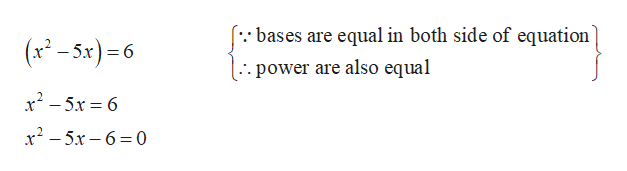# Solve the following exponential equation using properties of exponents. (Enter your answers as a comma-separated list. If an answer does not exist, enter DNE.)8x2 · 1/85x = 86 x=?

Question
78 views

Solve the following exponential equation using properties of exponents. (Enter your answers as a comma-separated list. If an answer does not exist, enter DNE.)

8x2 · 1/85x = 86

x=?
check_circle

star
star
star
star
star
1 Rating
Step 1

Given exponential equation

Step 2

To determine value of x by using exponential properties

Solution

Step 3

further calcu...help_outlineImage Transcriptionclose(*:: bases are equal in both side of equation (x-5x)=6 . power are also equal x2-5x6 x2-5x-6 0 fullscreen

### Want to see the full answer?

See Solution

#### Want to see this answer and more?

Solutions are written by subject experts who are available 24/7. Questions are typically answered within 1 hour.*

See Solution
*Response times may vary by subject and question.
Tagged in

### Functions